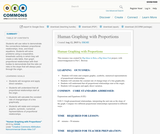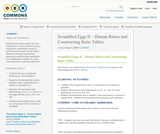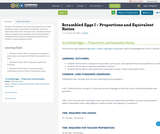# 6 Results

View
Selected filters:Conditions of Use:
Remix and Share
Rating

Students will explore multi-digit numbers and the relationship between ones, tens and hundreds; a digit in one place is 10x the digit in the place to its right. Students will use their bodies to represent digits in multi-digit numbers up to the hundredths place and compare these numbers using <, =, >. Students will use their bodies as multi-digit numbers to add and subtract.

Subject:
Mathematics
Material Type:
Activity/Lab
Teaching/Learning Strategy
Author:
01/28/2016Conditions of Use:
Remix and Share
Rating

Students will explore the concepts of place value using their bodies as tools. They will time themselves performing various kinesthetic tasks like jumping jacks and sit ups and use the numbers that they record from these activities in their exploration. Working in groups, they will practice adding and subtracting and comparing numbers. They will also come up with creative ways to represent numbers using the properties of operation and the rules of place value.

Subject:
Mathematics
Material Type:
Activity/Lab
Teaching/Learning Strategy
Author:
01/28/2016Conditions of Use:
Remix and Share
Rating

Students will explore the concepts of place value using their bodies as tools. They will time themselves performing various kinesthetic tasks like jumping jacks and sit ups and use the numbers that they record from these activities in their exploration. Working in groups, they will practice adding and subtracting and comparing numbers. They will also come up with creative ways to represent numbers using the properties of operation and the rules of place value.

Subject:
Mathematics
Material Type:
Activity/Lab
Teaching/Learning Strategy
Author:
01/28/2016Conditions of Use:
Remix and Share
Rating

Students will use ratios to demonstrate the connections between proportional relationships, lines, and linear equations. Students will solve problems using a cooperative, kinesthetic activity in which they will create a ratio table, then graph proportional relationships with their bodies to demonstrate that the ratio (or rate) is the slope that will always pass through the origin.

Subject:
Mathematics
Material Type:
Activity/Lab
Teaching/Learning Strategy
Author:
01/28/2016Conditions of Use:
Remix and Share
Rating

Students will analyze ratios and use proportions to solve problems using a cooperative, kinesthetic activity in which they will create “human ratios.” Students will apply proportional reasoning to demonstrate application of a multiplicative situation by using cross products to solving proportions.

Subject:
Mathematics
Material Type:
Activity/Lab
Teaching/Learning Strategy
Author:
01/28/2016Conditions of Use:
Remix and Share
Rating

Students will analyze ratios and use proportions to solve problems using a cooperative, kinesthetic activity in which they will create “human ratios.” Students will use ratios to compare two quantities, then solve problems cooperatively by demonstrating how proportions are written to show equivalent ratios.

Subject:
Mathematics
Material Type:
Activity/Lab
Teaching/Learning Strategy
Author: